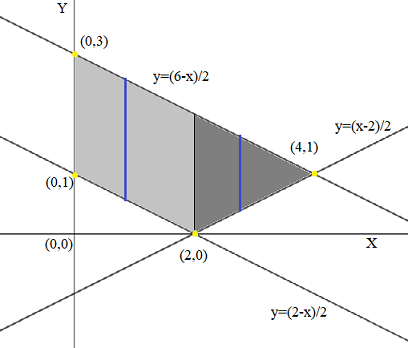# Let C be the trapezoid with vertices at (0, 1), (0, 3), (2, 0) and (4, 1). Show that \oint...

## Question:

Let C be the trapezoid with vertices at {eq}(0, 1), (0, 3), (2, 0) {/eq} and {eq}(4, 1) {/eq}. Show that

{eq}\oint (x-e^{y})dx-(xe^{y}-\arctan x)dy> 0 {/eq}

## Green's Theorem:

Consider a cyclic line integral of the form {eq}\displaystyle \oint_{C}(M(x,y) \ dx+N(x,y) \ dy) {/eq}. If the functions M and N are continuos functions of x and y respectively and if the partial derivatives {eq}\displaystyle \frac{\partial{M}}{\partial{y}} {/eq} and {eq}\displaystyle \frac{\partial{N}}{\partial{x}} {/eq} are also continuous, then the cyclic integral can be evaluated over the region R using the Green's theorem which states that,

$$\displaystyle \oint_{C}(M(x,y) \ dx+N(x,y) \ dy)=\iint_{R}\left(\frac{\partial{N}}{\partial{x}}- \frac{\partial{M}}{\partial{y}}\right) \ dx \ dy\\$$

Comapring the given integral to the standard form {eq}\displaystyle \oint_{C}(M(x,y) \ dx+N(x,y) \ dy) {/eq} we have the functions,

{eq}\displaystyle M(x,y)=(x-e^{y})\Rightarrow \boxed{\frac{\partial{M}}{\partial{y}}=-e^y}\\ \displaystyle N(x,y)=-(xe^{y}-\arctan x)\Rightarrow \boxed{\frac{\partial{N}}{\partial{x}}=-e^y+\frac{1}{1+x^2}} {/eq}

So according to the Green's theorem the integral can be evaluated by a double integral as follows,

{eq}\displaystyle \begin{align} \oint_{C}(M(x,y) \ dx+N(x,y) \ dy)&=\iint_{R}\left(\frac{\partial{N}}{\partial{x}}- \frac{\partial{M}}{\partial{y}}\right) \ dx \ dy\\ &=\iint_{R}\left[ \left( -e^y+\frac{1}{1+x^2}\right)-(-e^y)\right] \ dx \ dy\\ &=\iint_{R}\left[\frac{1}{1+x^2}\right] \ dx \ dy \end{align} {/eq}

Now the region R is the trapezoid as shown in the figure below.The region R is split up into two regions R1 (light grey) and R2 (dark grey) for ease of calculation of the double integral. SO the integral can now be evaluated as follows.

{eq}\displaystyle \begin{align} \iint_{R}\left[\frac{1}{1+x^2}\right] \ dy \ dx&=\int_{R_1}\left[\frac{1}{1+x^2}\right] \ dy \ dx+\int_{R_2}\left[\frac{1}{1+x^2}\right] \ dy \ dx\\ &=\int_{0}^{2}\int_{\frac{2-x}{2}}^{\frac{6-x}{2}}\left[\frac{1}{1+x^2}\right] \ dy \ dx+\int_{2}^{4}\int_{\frac{x-2}{2}}^{\frac{6-x}{2}}\left[\frac{1}{1+x^2}\right] \ dy \ dx\\ &=\int_{0}^{2}\left[\frac{y}{1+x^2}\right]_{\frac{2-x}{2}}^{\frac{6-x}{2}} \ dx + \int_{2}^{4}\left[ \frac{y}{1+x^2}\right]_{\frac{x-2}{2}}^{\frac{6-x}{2}} \ dx \\ &=\int_{0}^{2}\left[\left(\frac{6-x}{2(1+x^2)}\right)-\left(\frac{2-x}{2(1+x^2)} \right)\right] \ dx + \int_{2}^{4}\left[ \left( \frac{6-x}{2(1+x^2)}\right)-\left(\frac{x-2}{2(1+x^2)} \right)\right] \ dx\\ &=\int_{0}^{2}\left[ \frac{2}{1+x^2}\right] \ dx + \int_{2}^{4}\left[ \frac{4-x}{1+x^2}\right]\\ &=\left[ 2\arctan(x)\right]_0^2+\left[ 4\arctan(x)-\frac{\ln(x^2+1)}{2}\right]_2^4\\ &=2.2143+0.2628 & \text{ Uisng integration calculator }\\ &=2.4771\gt 0 \end{align} {/eq}